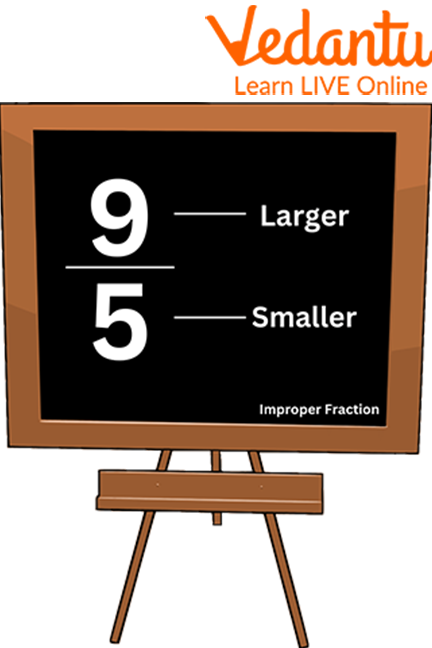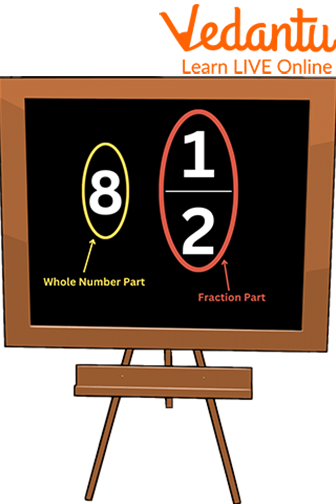Courses
Courses for Kids
Free study material
Free LIVE classes
More

# How to Convert Fractions Into Whole Numbers?LIVE
Join Vedantu’s FREE Mastercalss

## An Introduction to Fractions and Whole Numbers

Fractions represent any number of equal parts in a whole number. It is shown in the form of '/', as in, a/b. The number on the top is known as the ‘numerator’, and the number below is known as the ‘denominator’. Derived from the original Latin word 'Fractus,' the term ‘fraction’ means broken.

In the section on converting fractions, we'll go through how to convert improper fractions to whole or mixed numbers, improper fractions to mixed numbers, and whole numbers to improper fractions. Let’s get started on learning about it.

## Whole Numbers

Natural numbers along with zero (0) are referred to as whole numbers. We know that natural numbers refer to a set of counting numbers starting from 1, 2, 3, 4, and so on. Whole numbers are part of real numbers including all the positive integers and zero, but not fractions, decimals, or negative numbers.

The set of whole numbers in Mathematics is given as {0, 1, 2, 3, ...} which is denoted by the symbol W.

W = {0, 1, 2, 3, 4, …}

## Understanding Fraction Number

In Mathematics, fractions are represented as a numerical value, which defines a part of a whole. A fraction can be a portion or section of any quantity out of a whole, where the whole can be any number, a specific value, or a thing.

## Improper Fraction

An improper fraction is the type of fraction in which the numerator is more than or equal to its denominator. It is always the same or greater than the whole. For Example: $\dfrac{9}{5}$, $\dfrac{7}{3}$, $\dfrac{5}{2}$...etc.Improper Fraction

## Mixed Fraction

A mixed number, like $1 \dfrac{1}{3}$, is one that combines a whole number and a fraction. In this instance, the word "remainder" is used to describe the fraction $\dfrac{1}{3}$. A full number, on the other hand, is a number like 2, 3, and $4 .$Mixed Fraction

## How to Convert a Fraction Into a Whole Number

To convert a fraction into a whole number:

Divide the numerator by the denominator, only if the numerator is a multiple of the denominator.

Example: Convert $\dfrac{4}{2}$ into a whole number.

Ans: Here the numerator is 4 and the denominator is 2.

Since we know that to make this fraction into a whole number, the numerator should be a multiple of the denominator.

Here, 4 is the multiple of 2.

Therefore, we can write $\dfrac{4}{2}=2$

Hence, $\dfrac{4}{2}$ into a whole number is equal to 2.

## Converting Improper Fractions Into Whole Numbers

The following are the steps to convert improper fractions into whole numbers:

Step 1: Verify if the fraction's numerator is bigger than or equal to the denominator by looking at the fraction's numerator.

For Example, $\dfrac{24}{12}$, where the numerator is 24 and the denominator is 12. So, the numerator is greater than the denominator.

Step 2: Now, we can change our improper fraction into a whole number if the above condition follows.

If the numerator is larger than the denominator, divide it and check the remainder. As there should not be any remainder left.

For instance, if the improper fraction is $\dfrac{24}{12}$ perfectly divided by 12, leaving no remainder, and gives the result as 2.

Note: The fraction cannot be converted into a whole number if there is a remainder when the numerator and denominator are divided.

## Solved Examples

Q1. Convert the whole number “10” into a fraction.

Ans: Given that the whole number is 10

Multiply and divide the number by 1

$n=10 \times \dfrac{1}{1}$

$n=\dfrac{10}{1}$

Hence, the fraction is $\dfrac{10}{1}$

Q2. Convert $\dfrac{27}{18}$ into a mixed number.

Ans: Divide the numerator by the denominator:

$27 \div 18=1$ with the remainder of 9.

Now, write down the whole number 1 and then write down the rem numerator (9) above the denominator (18) as:

$1 \dfrac{9}{18}$

You can reduce the fraction part of this mixed number :

Find the greatest common factor of 9 and 18

$\operatorname{GCF}(9,18)=9$

$\dfrac{9 \div 9}{18 \div 18}=\dfrac{1}{2}$

Therefore, the improper fraction $\dfrac{27}{18}$ converted to a mixed number is: $1 \dfrac{1}{2}$.

Q3. Simplify improper fractions $\dfrac{8}{6}+\dfrac{10}{6}$ and find out whether the result is a whole number or not.

Ans: $\dfrac{8}{6}+\dfrac{10}{6}$

Here the same denominator is $6 .$

$=\dfrac{8}{6}+\dfrac{10}{6}$

$=\dfrac{(8+10)}{6}$

$=\dfrac{18}{6}$

Here $\dfrac{18}{6}$ is an improper fraction after further simplifying,

$=\dfrac{18}{6}=3$

## Practice Questions

Q1. Change the whole number 6 into an improper fraction.

Ans: $\dfrac{36}{6}$

Q2. Change $\dfrac{16}{8}$ into a whole number.

Ans: $2 .$

Q3. Convert $\dfrac{34}{8}$ into a mixed number.

Ans: $4 \dfrac{1}{4}$

## Summary

We turn the fraction into an operational version of the mathematical expression in order to deal with expressions such as those that are improper. However, the majority of improper fractions are transformed into mixed numbers with a remainder. Some improper fractions can be transformed into whole numbers. A fraction can only be expressed as a whole number when the numerator is a multiple of the denominator. If there is a remainder after dividing the numerator and denominator, the fraction cannot be expanded into a whole number.

Last updated date: 01st Oct 2023
Total views: 92.4k
Views today: 1.92k

## FAQs on How to Convert Fractions Into Whole Numbers?

1. Does a fraction always have to be a whole number?

It is possible for a fraction to have a reciprocal that is either a fraction or a whole number.

2. What do you call fractions with the same denominator?

Fractions with the same denominator are known as like fractions. For example, $\dfrac{4}{7}$, $\dfrac{3}{7}$, $\dfrac{5}{7}$ are like fractions because they have the same denominator.

3. How are fractions utilised in everyday life?

To determine how much of an ingredient to use, bakers use fractions. Each minute is a fraction of an hour, thus we use fractions to tell the time. And when there is a sale, fractions are utilised to calculate discounts.